﻿ An Alternative Proof to Markowitz’s Model

### An Alternative Proof to Markowitz’s Model

Yaniv ZaksOPEN ACCESSPEER-REVIEWED

## An Alternative Proof to Markowitz’s Model

Department of Mathematics, Bar-Ilan University, Israel

### Abstract

In the fundamental paper on portfolio selection, Markowitz (1952) described via geometric reasoning his innovative theory and provided the explicit optimal selection for the cases of 3 and 4 assets. Merton (1972) obtained for the general case the efficient portfolio frontiers explicitly by using Lagrange multipliers. In this paper, we suggest a geometric approach to achieve the explicit optimal selection for the general case thus generalizing Markowitz’s original approach to achieve the explicit presentation of the desired selection.

• Zaks, Yaniv. "An Alternative Proof to Markowitz’s Model." Journal of Finance and Economics 1.3 (2013): 33-35.
• Zaks, Y. (2013). An Alternative Proof to Markowitz’s Model. Journal of Finance and Economics, 1(3), 33-35.
• Zaks, Yaniv. "An Alternative Proof to Markowitz’s Model." Journal of Finance and Economics 1, no. 3 (2013): 33-35.

 Import into BibTeX Import into EndNote Import into RefMan Import into RefWorks

### 1. Introduction

The basis of the portfolio selection problem was first defined by the Nobel laureateHarry Markowitz in 1952. In his famous paper he combined,for the first time, the mean and the variance of the return in order to select an optimal portfolio. The variance stands for measuring the risk of the portfolio. The Nobel laureate Merton H. Miller refered in his essay "The history of finance: an eyewitness account" to Markowitz's model and wrote "Markowitz in this remarkable paper gave, for the first time, a precise definition of what had hitherto been just vague buzzwords: risk and return". Thus, it is not surprising that Markowitz's model is the first model that every student learns in the field of portfolio selection. Many times this is also the only model that is studied.

In this paper, we give an alternative proof to Markowitz's model. Markowitz (1952) explained his innovative theory by geometric reasoning and provided the explicit optimal selection for the cases of 3 and 4 assets. Twenty years later, Merton (1972) used the Lagrange multipliers method to obtain an explicit formula for the efficient portfolio frontier for the general case. Solving the Lagrange first order condition gives us a critical point that might be the desired minimum, but theoretically it might be a maximum or a saddle point as well. While teaching this model in the classroom, there is usually no discussion as to the character of the critical point. In order to verify that we have obtained a minimum, we should verify that some extra conditions hold. Unfortunately, this kind of discussion is very rare in the classroom due to its complexity.

We suggest a geometric approach to achieve the explicit optimal portfolio for the general case; thus we follow Markowitz's original presentation of his theory. Following the geometric approach, it is easy to explain that there exist a solution and to show that this solution is unique. To derive the optimal solution we consider well-known formulas in analytic geometry.

It is important to mention that in our analysis it is essential to assume that the covariance marix is a positive definite matrix. This assumption is required by Merton as well. The two techniques, obviously, lead to the same single solution. According to the method we are using to solve the model in this paper, there is no need for extra calculations or explanations in order to verify that the obtained solution is the minimum. This is the major advantage of our approach over the Lagrange multipliers method.

We will present the objective function of Markowitz's model in terms of a Euclidean projection of a point on a hyperplane. A hyperplane of an n-dimensional space is a subspace with dimension n-1, for example, a line in a plane (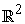) or a plane in. A hyperplane is determined by a vector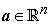and a scalar b. In mathematical notations, a hyperplane is defined as the following set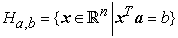whereis the inner product of x and a, i.e.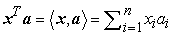. Note that for any two vectors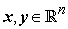we use the notations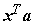andalternately throughout the rest of the paper.

Let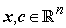. The Euclidian distance between x and c is denoted by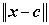such that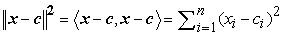A Euclidean projection of a point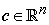on the hyperplaneis a point that belongs to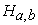that is closest to the point c (in the sense of Euclidean distance). It is well known in analytic geometry that such a point exists and it is unique. Moreover, there is an explicit solution for the projection. In mathematical notations, we state the problem of finding the Euclidian projection as the following optimization problem:and the optimal solution, i.e., the projection, is: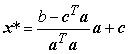(1)

### 2. The Optimal Solution for Markowitz Model

The Markowitz model takes into consideration the expected valueand the varianceof a portfolio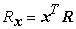, where R is the vector of returns and x is the weights vector of the portfolio. The optimization problem in the Markowitz model is the following:(2)

where τ is the risk tolerance of the investor.

It is well known (Panjer et al. (2001), Best & Grauer (1992)) that the optimal solution is the following: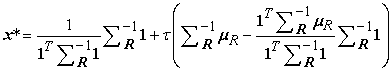(3)

Where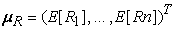is the vector of expectations and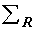is the covariance matrix of the returns. Furthermore,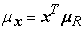and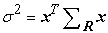. By changing the parameter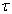, we obtain the efficient frontier.In our analysis we refer to the equivalent minimization problem: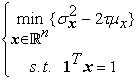(4)

The covariance matrixis a symmetric matrix and we assume that it is a positive definite matrix as well. Hence there exists a matrix G such that,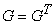and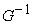exists. It follows that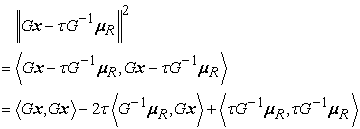Hence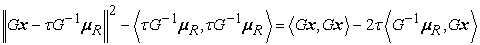(5)

By the equality, it follows that the first term on the right hand side satisfies:(6)

and the second term satisfies:(7)

Hence, the following equality holds:where the last term on the right hand side does not depend on x. Therefore, the optimization problem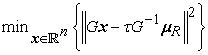has the same optimal solution asIt remains to solve the following optimization problem: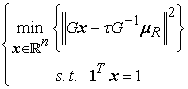(8)

Let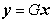. Substituting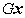by y in(8) leads to: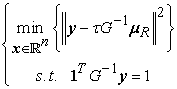(9)

By the symmetry of G, it is possible to write the constraint as. We obtain that the optimization problem in (9) is equivalent to the problem of finding the Euclidean projection of the vectoronto the hyperplane. This problem has the unique solution: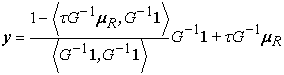(10)

Notice thatand. Substitutingin(10) yields: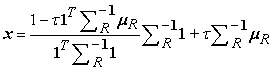(11)

as required. Note that in the above proof, we did not consider any derivatives.

### 3. Conclusions

In this paper, we solved the Markowitz's model by following a geometric approach. We showed that the original optimization problem is equivalent to the problem of finding a Euclidean projection of a point on a hyperplane. For this reason, there was no need for any further calculations in order to verify whether or not this solution leads to the minimum of the objective function. This is the main advantage of this technique over the Lagrange multipliers method.

The proposed method may be valuable in some generalizations as well. One generalization of Markowitz model is presented in Kriens &van Lieshout (1998). In that paper, the authors replaced the expectation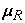and the covariance matrixby the expectation and the covariance matrix of a non-linear transformation of the random vector R. They required that the new expectation and the new variance be concave and continuously differential on the set of feasible portfolios. Following the technique in this paper, we may consider any transformation that keeps the positive definite property of the covariance matrix. In addition, any transformation of the expectation is allowed. Another generalization appears in Li & Ng(2000), where the authors are seeking the optimal capital allocation in a multiperiod portfolio. Following our technique, it is easy to explain the existence of a single optimal solution.

### References

  Kriens J. and van Lieshout J. Th., 1998. Notes on the Markowitz portfolio selection method. Statistica Neerlandica 42 (3): 181-191.In article CrossRef  Li D., Ng W.L., 2000, Optimal dynamic portfolio selection: multiperiod mean-variance formulation, Mathematical Finance 10(3): 387-406.In article CrossRef  Markowitz H., 1952. Portfolio selection. The Journal ofFinance 7 (1): 77-91.In article  Merton R.C., 1972. An analytic derivation of the efficient portfolio frontier. Journal of Financial and Quantitative Analysis 7 (4): 1851-72.In article CrossRef  Best M.J. and Grauer R.R., 1992. Positively weighted minimum-variance portfolios and the structure of asset expected returns. Journal of Financial and Quantitative Analysis 27: 513-537.In article CrossRef  Miller M.H., 2000, The history of finance: an eyewitness account, Journal of Applied Corporate Finance 13(2): 8-14.In article CrossRef  Panjer H.H., editor: Boyle P.P....[et al.], 2001, Financial Economics: With Application to Investments, Insurance and Pensions. The Society of Actuaries.In article